SLUSC89 November   2015

PRODUCTION DATA.

1. Features
2. Applications
3. Description
4. Revision History
5. Pin Configuration and Functions
6. Specifications
7. Detailed Description
1. 7.1 Overview
2. 7.2 Functional Block Diagram
3. 7.3 Feature Description
4. 7.4 Device Functional Modes
8. Application and Implementation
1. 8.1 Application Information
2. 8.2 Typical Application
1. 8.2.1 Design Requirements
2. 8.2.2 Detailed Design Procedure
3. 8.2.3 Application Curves
9. Power Supply Recommendations
10. 10Layout
11. 11Device and Documentation Support
1. 11.1 Device Support
2. 11.2 Documentation Support
3. 11.3 Community Resources
5. 11.5 Electrostatic Discharge Caution
6. 11.6 Glossary
12. 12Mechanical, Packaging, and Orderable Information

• DRC|10
• DRC|10

## 8 Application and Implementation

NOTE

Information in the following applications sections is not part of the TI component specification, and TI does not warrant its accuracy or completeness. TI’s customers are responsible for determining suitability of components for their purposes. Customers should validate and test their design implementation to confirm system functionality.

### 8.1 Application Information

The TPS40210-EP is a 4.5-V to 52-V low-side controller with an integrated gate driver for a low-side N-channel MOSFET. This device is typically used in a boost topology to convert a lower DC voltage to a higher DC voltage with a peak current limit set by an external current sense resistor. It can also be configured in a SEPIC, Flyback and LED drive applications. In higher current applications, the maximum current can also be limited by the thermal performance of the external MOSFET and rectifying diode switch. Use the following design procedure to select external components for the TPS40210-EP. The design procedure illustrates the design of a typical boost regulator with the TPS40210-EP. Alternatively, use the WEBENCH software to generate a complete design. The WEBENCH software uses an iterative design procedure and accesses a comprehensive database of components when generating a design.

### 8.2 Typical Application

The following example illustrates the design process and component selection for a 12-V to 24-V nonsynchronous boost regulator using the TPS40210-EP controller.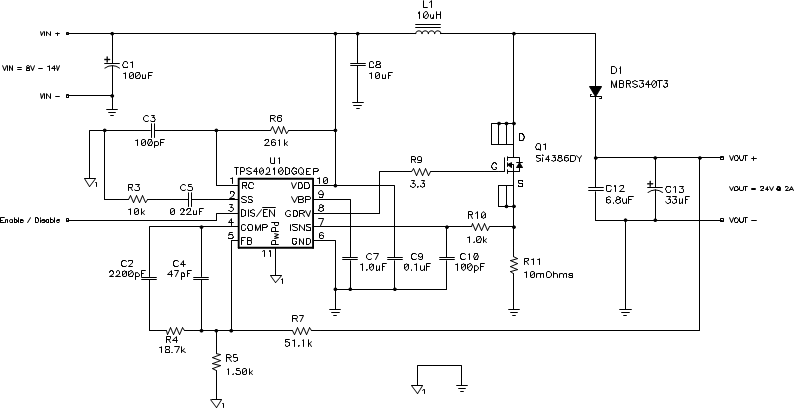Figure 29. TPS40210-EP Design Example – 12-V to 24-V at 2 A

### Table 1. TPS40210-EP Design Example Specifications

PARAMETER TEST CONDITIONS MIN TYP MAX UNIT
INPUT CHARACTERISTICS
VIN Input voltage 8 12 14 V
IIN Input current 4.4 A
VIN(UVLO) Input undervoltage lockout 4.5 V
OUTPUT CHARACTERISTICS
VOUT Output voltage 23.5 24.0 24.5 V
Line regulation 1%
VOUT(ripple) Output voltage ripple 500 mVPP
IOUT Output current 8 V ≤ VIN ≤ 14 V 0.1 1 2.0 A
IOCP Output overcurrent inception point 3.5
Transient response
Overshoot threshold voltage 500 mV
Settling time 5 ms
SYSTEM CHARACTERISTICS
ƒSW Switching frequency 600 kHz
ηPK Peak efficiency VIN = 12 V 95%
η Full load efficiency VIN = 12 V, IOUT = 2 A 94%
TOP Operating temperature range 8 V ≤ VIN ≤ 14 V, IOUT ≤ 2 A 25 °C
MECHANICAL DIMENSIONS
W Width 1.5 inch
L Length 1.5
h Height 0.5

#### 8.2.2.1 Duty Cycle Estimation

The duty cycle of the main switching MOSFET is estimated using Equation 31 and Equation 32.

Equation 31.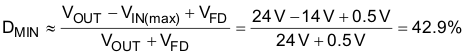Equation 32.Using an estimated forward drop (VFD) of 0.5V for a schottky rectifier diode, the approximate duty cycle is 42.9% (minimum) to 67.3% (maximum).

#### 8.2.2.2 Inductor Selection

The peak-to-peak ripple is chosen to be 30% of the maximum input current.

Equation 33.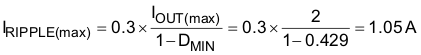The minimum inductor size can be estimated using Equation 34.

Equation 34.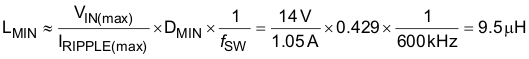The next higher standard inductor value of 10 μH is selected. The ripple current for nominal and minimum VIN is estimated by Equation 35 and Equation 36.

Equation 35.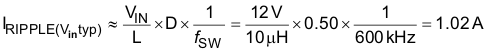Equation 36.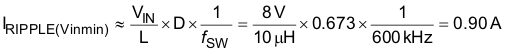The worst case peak-to-peak ripple current occurs at 50% duty cycle (VIN = 12.25 V) and is estimated as 1.02 A. Worst case RMS current through the inductor is approximated by Equation 37.

Equation 37.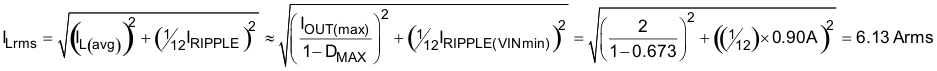The worst case RMS inductor current is 6.13 A. The peak inductor current is estimated by Equation 38.

Equation 38.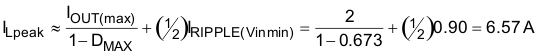A 10-μH inductor with a minimum RMS current rating of 6.13 A and minimum saturation current rating of 6.57 A must be selected. A TDK RLF12560T-100M-7R5 7.5-A 10-μH inductor is selected.

This inductor power dissipation is estimated by Equation 39.

Equation 39.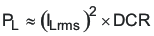The TDK RLF12560T-100M-7R5 12.4-mΩ DCR dissipates 466-mW of power.

#### 8.2.2.3 Rectifier Diode Selection

A low forward voltage drop schottky diode is used as a rectifier diode to reduce its power dissipation and improve efficiency. Using 80% derating on VOUT for ringing on the switch node, the rectifier diode minimum reverse break-down voltage is given by Equation 40.

Equation 40.The diode must have reverse breakdown voltage greater than 30 V. The rectifier diode peak and average currents are estimated by Equation 41 and Equation 42.

Equation 41.Equation 42.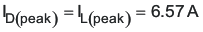The power dissipation in the diode is estimated by Equation 43.

Equation 43.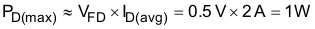For this design, the maximum power dissipation is estimated as 1 W. Reviewing 30-V and 40-V schottky diodes, the MBRS340T3, 40-V, 3-A diode in an SMC package is selected. This diode has a forward voltage drop of 0.48 V at 6 A, so the conduction power dissipation is approximately 960 mW, less than half its rated power dissipation.

#### 8.2.2.4 Output Capacitor Selection

Output capacitors must be selected to meet the required output ripple and transient specifications.

Equation 44.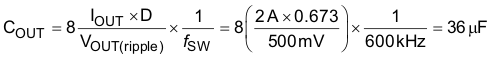Equation 45.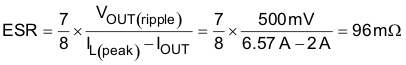A Panasonic EEEFC1V330P 35-V 33-μF, 120-mΩ bulk capacitor and a 6.8-μF ceramic capacitor are selected to provide the required capacitance and ESR at the switching frequency. The combined capacitance of 39.8 μF and ESR of 60 mΩ are used in compensation calculations.

#### 8.2.2.5 Input Capacitor Selection

Since a boost converter has continuous input current, the input capacitor senses only the inductor ripple current. The input capacitor value can be calculated by Equation 46 and Equation 47 .

Equation 46.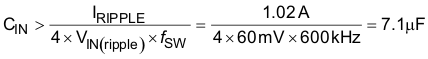Equation 47.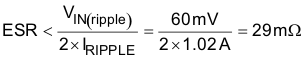For this design to meet a maximum input ripple of 60mV (1/2% of VIN nominal), a minimum 7.1-μF input capacitor with ESR less than 29mΩ is needed. A 10-μF, X7R ceramic capacitor is selected.

#### 8.2.2.6 Current Sense and Current Limit

The maximum allowable current sense resistor value is limited by both the current limit and sub-harmonic stability. These two limitations are given by Equation 48 and Equation 49.

Equation 48.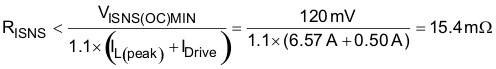Equation 49.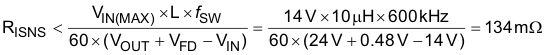With 10% margin on the current limit trip point (the 1.1 factor) and assuming a maximum gate drive current of 500 mA, the current limit requires a resistor less than 15.4 mΩ and stability requires a sense resistor less than 134 mΩ. A 10-mΩ resistor is selected. Approximately 2 mΩ of routing resistance is added in compensation calculations.

The power dissipation in RISNS is calculated by Equation 50.

Equation 50.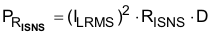At maximum duty cycle, this is 0.253 W.

#### 8.2.2.7 Current Sense Filter

To remove switching noise from the current sense, an RC filter is placed between the current sense resistor and the ISNS pin. A resistor with a value between 1 kΩ and 5 kΩ is selected and a capacitor value is calculated by Equation 51.

Equation 51.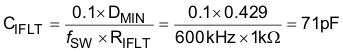For a 1-kΩ filter resistor, 71pF is calculated and a 100-pF capacitor is selected.

#### 8.2.2.8 Switching MOSFET Selection

The TPS40210-EP drives a ground referenced N-channel FET. The RDS(on) and gate charge are estimated based on the desired efficiency target.

Equation 52.For a target of 95% efficiency with a 24-V Input voltage at 2 A, maximum power dissipation is limited to 2.526 W. The main power dissipating devices are the MOSFET, inductor, diode, current sense resistor and the integrated circuit, the TPS40210-EP.

Equation 53.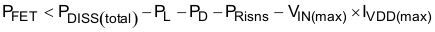This leaves 812 mW of power dissipation for the MOSFET. This can likely cause an SO-8 MOSFET to get too hot, so power dissipation is limited to 500 mW. Allowing half for conduction and half for switching losses, we can determine a target RDS(on) and QGS for the MOSFET by Equation 54 and Equation 55.

Equation 54.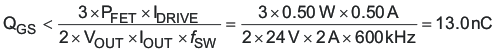A target MOSFET gate-to-source charge of less than 13.0 nC is calculated to limit the switching losses to less than 250 mW.

Equation 55.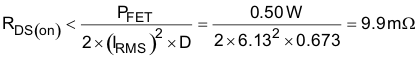A target MOSFET RDS(on) of 9.9 mΩ is calculated to limit the conduction losses to less than 250 mW. Reviewing 30-V and 40-V MOSFETs, an Si4386DY 9-mΩ MOSFET is selected. A gate resistor was added per Equation 30. The maximum gate charge at VGS= 8V for the Si4386DY is 33.2 nC, this implies RG = 3.3 Ω.

#### 8.2.2.9 Feedback Divider Resistors

The primary feedback divider resistor (RFB) from VOUT to FB should be selected between 10 kΩ and 100 kΩ to maintain a balance between power dissipation and noise sensitivity. For a 24-V output a high feedback resistance is desirable to limit power dissipation so RFB = 51.1 kΩ is selected.

Equation 56.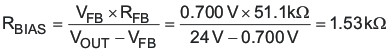RBIAS = 1.50kΩ is selected.

#### 8.2.2.10 Error Amplifier Compensation

Compensation selection can be done with aid of WEBENCH to select compensation components or with the aid of the average Spice model to simulate the open loop modulator and power stage gain. Alternatively the following procedure gives a good starting point.

While current mode control typically only requires Type II compensation, it is desirable to layout for Type III compensation to increase flexibility during design and development. Current mode control boost converters have higher gain with higher output impedance, so it is necessary to calculate the control loop gain at the maximum output impedance, estimated by Equation 57.

Equation 57.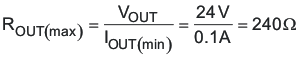The transconductance of the TPS40210-EP current mode control can be estimated by Equation 58.

Equation 58.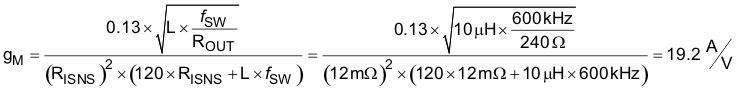The maximum output impedance ZOUT, can be estimated by Equation 59.

Equation 59.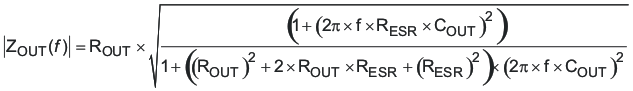Equation 60.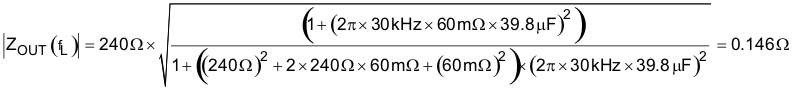At the desired crossover frequency (ƒL) of 30 kHz, ZOUT becomes 0.146 Ω.

The modulator gain at the desired cross-over can be estimated by Equation 61.

Equation 61.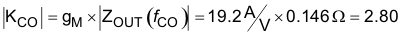The feedback compensation network needs to be designed to provide an inverse gain at the cross-over frequency for unity loop gain. This sets the compensation mid-band gain at a value calculated in Equation 62.

Equation 62.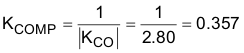To set the mid-band gain of the error amplifier to KCOMP use Equation 63.

Equation 63.R4 = 18.7 kΩ selected.

Place the zero at 1/10th of the desired cross-over frequency.

Equation 64.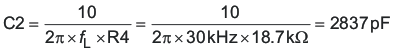C2 = 2200 pF selected.

Place a high-frequency pole at about 5 times the desired cross-over frequency and less than one-half the unity gain bandwidth of the error amplifier:

Equation 65.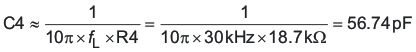Equation 66.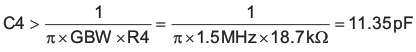C4 = 47 pF selected.

#### 8.2.2.11 RC Oscillator

The RC oscillator calculation is given as shown in Equation 14 in the datasheet, substituting 100 for CT and 600 for ƒSW. For a 600-kHz switching frequency, a 100pF capacitor is selected and a 262-kΩ resistor is calculated (261-kΩ selected).

#### 8.2.2.12 Soft-Start Capacitor

Since VDD > 8 V, the soft-start capacitor is selected by using Equation 67 to calculate the value.

Equation 67.For TSS = 12 ms, CSS = 240 nF. A 220-nF capacitor is selected.

#### 8.2.2.13 Regulator Bypass

A regulator bypass (BP) capacitor of 1.0 μF is selected per the datasheet recommendation.

### Table 2. Bill of Materials

REFERENCE
DESIGNATOR
DESCRIPTION SIZE PART
NUMBER
MANUFAC-
TURER
C1 100 μF, aluminum capacitor, SM, ± 20%, 35 V 0.406 x 0.457 EEEFC1V101P Panasonic
C2 2200 pF, ceramic capacitor, 25 V, X7R, 20% 0603 Std Std
C3 100 pF, ceramic capacitor, 16 V, C0G, 10% 0603 Std Std
C4 47 pF, ceramic capacitor, 16V, X7R, 20% 0603 Std Std
C5 0.22 μF, ceramic capacitor, 16 V, X7R, 20% 0603 Std Std
C7 1.0 μF, ceramic capacitor, 16 V, X5R, 20% 0603 Std Std
C8 10 μF, ceramic capacitor, 25 V, X7R, 20% 0805 C3225X7R1E106M TDK
C9 0.1 μF, ceramic capacitor, 50 V, X7R, 20% 0603 Std Std
C10 100 pF, ceramic capacitor, 16 V, X7R, 20% 0603 Std Std
D1 Schottky diode, 3 A, 40 V SMC MBRS340T3 On Semi
L1 10 μH, inductor, SMT, 7.5 A, 12.4 mΩ 0.325 x 0.318 inch RLF12560T-100M-7R5 TDK
Q1 MOSFET, N-channel, 40 V, 14 A, 9mΩ SO-8 Si4840DY Vishay
R3 10 kΩ, chip resistor, 1/16 W, 5% 0603 Std Std
R4 18.7 kΩ, chip resistor, 1/16 W, 1% 0603 Std Std
R5 1.5 kΩ, chip resistor, 1/16 W, 1% 0603 Std Std
R6 261 kΩ, chip resistor, 1/16 W, 1% 0603 Std Std
R7 51.1 kΩ, chip resistor, 1/16 W, 1% 0603 Std Std
R9 3.3 Ω, chip resistor, 1/16 W, 5% 0603 Std Std
R10 1.0 kΩ, chip resistor, 1/16 W, 5% 0603 Std Std
R11 10 mΩ, chip resistor, 1/2 W, 2% 1812 Std Std
U1 IC, 4.5 V-52 V I/P, current mode boost controller DGQ10 TPS40210-EPDGQ TI

#### 8.2.3 Application Curves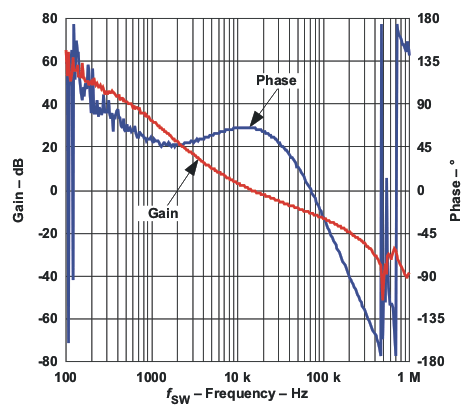Figure 30. Gain and Phase vs Frequency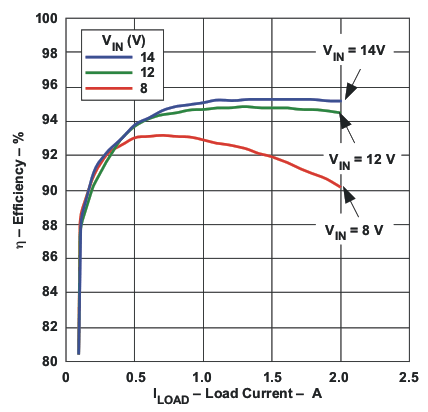Figure 32. Efficiency vs Load Current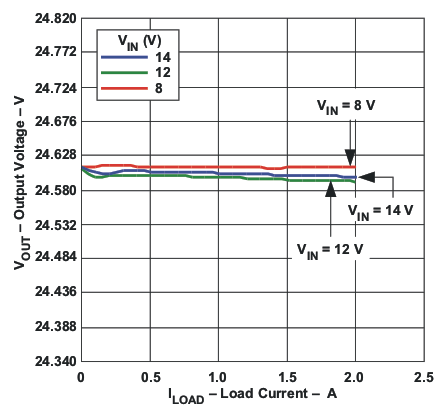Figure 34. Output Voltage vs Load Current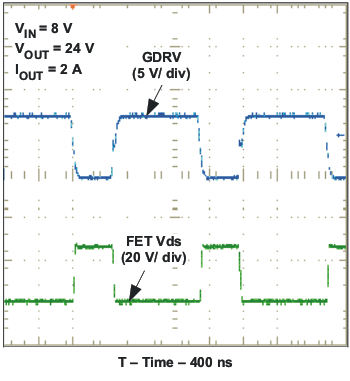Figure 31. FET VDS and VGS Voltages vs Time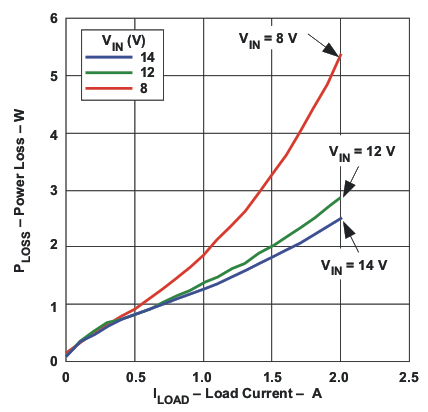Figure 33. Power Loss vs Load Current